## Feedback Amplifier Stability

Aug 04, 2012

### Introduction:

This note discusses operational amplifier feedback-network stability. The feedback network discussed here consists of two parallel RC combinations in the feedback loop as shown below. This simplified but practical configuration is used to illustrate several examples of feedback stability:To focus on the effects of this simplified feedback network, the operational amplifier will be idealized with zero output impedance, input impedances that can be lumped into Ri, Ci and a single pole Open Loop Gain characteristic:Irrespective of the actual circuit configuration (non-inverting amplifier, inverting amplifier, transimpedance amplifier etc.), the circuit stability (against oscillation) is determined solely by the properties of this complex voltage-divider feedback network between the operational amplifier output and the inverting (-) input of the amplifier.

The circuit will be stable against oscillation provided that the Noise-Gain magnitude curve intersects the operational amplifier Open-Loop Gain magnitude curve so that the phase shift "around the loop" at this intersection is greater than approximately 45 degrees and preferably greater than 60 degrees. In principal, the phase margin must be reduced to 0 degrees for instability (positive feedback) but there will always be other cumulative phase shifts. The 45-60 degrees is usually a safe practical margin for ensuring stability. This is equivalent to stating that the relative rate of intersection of the noise-gain and open-loop gain curves must be 20dB/decade or less.

The frequency-dependent Noise Gain which is the inverse of the complex feedback fraction beta, for this specific feedback network is given by: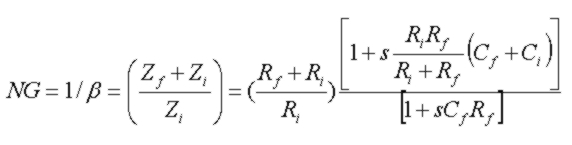where the feedback-network impedances, Zf and Zi for this specific configuration of parallel R, C components are: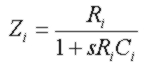The initial factor (Rf + Ri)/Ri is the DC noise gain. The complex frequency is:At high frequency, the noise gain approaches (1 + Ci/Cf), independent of the resistors in the divider network. The noise gain contains one zero and one pole as can be seen when rewritten as:or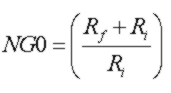The noise-gain zero frequency is given by: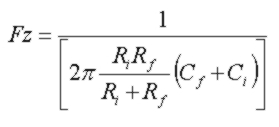and the noise-gain pole frequency is given by: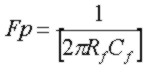The point of intersection in frequency of the noise gain (magnitude) with the open-loop gain (magnitude) is given by a simple quadratic equation for fc^2: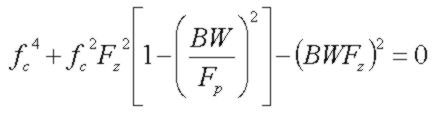where BW = GBW/NG0

The phase angle of the loop gain, Ao(fc)*beta(fc)) or equivalently Ao(fc)/Noise-Gain(fc)), at this intersection point determines the circuit stability and is given by: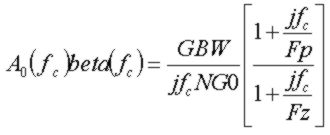As the phase angle of the loop gain (phase shift from the output to the inverting input) approaches -180 degrees, the feedback will become positive and the circuit will oscillate. The Phase Margin is defined as the phase "headroom" available between this oscillation point and the actual loop-gain phase shift at the intersection frequency, or: Phase Margin = 180 + phase(Noise-Gain(fc))

### Discussion and Examples:

Generally speaking, the noise-gain pole frequency Fp, the noise-gain zero frequency Fz and the intersection frequency fc can appear in any order in frequency, depending on the GBW of the operational amplifier and the magnitudes of Cf, Rf, Ci, Ri. To illustrate this, the first example below shows a configuration which would apply to a transimpedance amplifier (with very high Ri and therefore a low-frequency gain of unity or 0dB). With component values shown, the phase margin is very small at 25 degrees and the circuit will almost certainly oscillate. Another way of stating this is that the noise-gain curve approaches the intersection to the open-loop gain curve at a rate of close to 40 dB/decade. This arises due to the low frequency of the noise-gain zero, Fz due to the fairly high input capacitance. Since the Fz occurs at a much lower frequency than Fp, the noise gain will initially increase as shown which gradually lowers the phase margin. If the pole frequency Fp can be LOWERED by increasing the feedback shunt capacitance Cf, this will tend to flatten the noise-gain curve before intersection with the open-loop gain curve, leading to better phase margin. Alternately, a higher GBW amplifier could be used (say 100 MHz) which, with the same component values, would move the intersection point to higher frequency where the noise-gain curve has flattened out as a result of the pole "cancelling out" the shift due to Fz.The second example below shows a configuration which would apply typically to a moderately high gain (~ 30dB) non-inverting amplifier configuration. Note that in this case, the order of the noise-gain zero and pole are reversed compared to the first example and also the intersection point fc (14 MHz) occurs at a higher frequency than either Fz or Fp. In this example, Fp and Fz are fairly close together in frequency and since the pole Fp occurs at lower frequency, the noise gain decreases initially until the zero at Fz cancels the phase shift accumulation of the pole and flattens out the noise-gain curve reasonably well before the intersection point. In this case the phase margin at fc is 116 degrees, a very stable configuration. Increasing Cf will move Fp to lower frequency resulting in a greater drop in noise gain before Fz can flatten the gain. This is typically used to lower the gain of amplifiers where extra bandwidth is not required. Note however that the noise gain (magnitude) will always be greater than unity (0 dB). Also, if the operational amplifier is NOT unity gain stable (the single pole Ao(s) open loop gain curve discussed here is always unity gain stable), then the noise gain must not be lowered below the minimum stable gain value at the intersection point fc.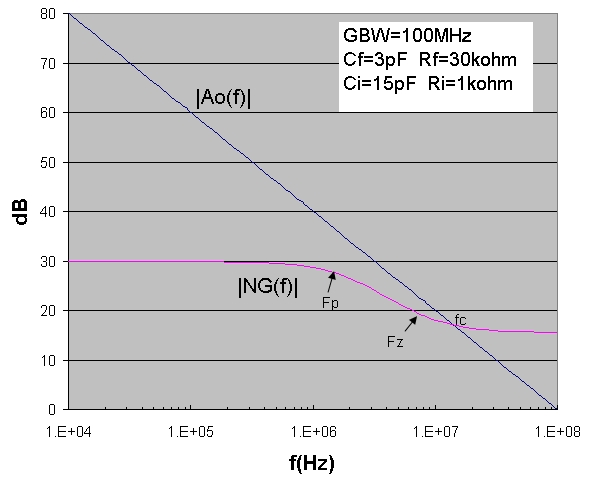An example for a GBW=12 MHz transimpedance amplifier demonstrates how the noise gain, transimpedance and phase margin change as the feedback capacitance is continuously changed.

### Feedback Stability, Bandwidth and Noise Calculator:

An accurate calculator is provided below that computes feedback amplifier properties for given GBW, Rf, Cf, Ri and Ci in the feedback circuit described above. The circuit and op-amp model used for this calculator are:
• op-amp: finite GBW with single-pole Ao(s); input capacitances (include in Ci); op-amp internal output resistance = 0
• feedback resistance Rf
• feedback total shunt capacitance Cf
• input total shunt resistance Ri at inverting input
• input total shunt capacitance Ci at inverting input
The calculated stability properties are:
• noise-gain zero Fz and pole Fp frequencies
• intersection frequency Fc where |Ao(s)beta(s)|=1
• phase Margin at Fc
• noise gain value |NG(Fc)| at intersection frequency
The f3dB bandwidth, Q factor, and the peaking frequency Fpeak and peaking amplitude Peak are calculated if peaking occurs, for the transimpedance (Tz) and inverting (INV) amplifier configurations. If it exists, the Cf value which produces the maximally flat Tz response (for Q = 1/Sqrt(2)) is calculated. The parameters are the same for the Tz and INV circuits since the transfer function for the INV circuit differs from the Tz circuit by a constant factor of 1/Ri. This can also be seen easily by noting that the INV circuit input voltage Vi in series with Ri can be Thevenized into an equivalent fixed current source Ip = Vi/Ri in parallel with the same resistance Ri and Ci, identical to the Tz circuit with the same feedback network values.
Total input-referred noise current is calculated (see discussion below) for the Tz configuration for the conditions described below.
For the noninverting (NI) circuit configuration, the f3db bandwidth, peaking frequency and values are also calculated (different then Tz/Inv configurations). The text area below the calculator summarizes results cumulatively which can be conveniently cut/pasted for future reference. These three circuits are shown below along with the transfer functions for the specific feedback network discussed above.

The default parameters for the calculator demonstrate a transimpedance amplifier example with a high gain (5Mohm) and total input capacitance (20 pF total) with Cf chosen to be close to the maximally flat value.. This example would apply for example to the 16 MHz OPA627 DiFet op-amp used with a 5 pF small area photodiode such as the Vishay Semiconductors BPW24R Si photodiode:

To use the calculator, enter the values for GBW (in MHz), Rf, Ri (in kohms), Cf, Ci (in pF) and spectral densities for the op-amp voltage noise en and current noise in and click "Get Parameters". The parameters are updated and results are added to the text area:

Feedback Stability, Bandwidth and Noise Calculator
GBW (MHz):      en (nV/√Hz):      in (pA/√Hz):
Rf (k):     Cf (pF):      Ri (k):     Ci (pF):
Noise Gain Stability Properties   Fz (Hz):   Fp (Hz):
Fc (Hz):   Margin (deg):
NG (Fc):
Tz and INV Circuit Properties   f3dB (Hz):   Q:
Fpeak (Hz):   Peak (dB):
Cf(flat) (pF):
Tz Noise
(in bandwidth Fp)
n_in (pA/√Hz):   n_Rf (pA/√Hz):
n_en (pA/√Hz):   n_en+ (pA/√Hz):
In_eq (pA/√Hz):
In_tot (nA):   Vn_tot (uV):
NI Circuit Properties   f3dB (Hz):
Fpeak (Hz):   Peak (dB):

The same calculator is also available separately on a simplified page.
A alternate calculator with more detailed noise results is also available.Fz: Noise gain zero frequency
Fp: Noise gain pole frequency
Fc: Intersection frequency of noise gain and open loop gain
Margin: Phase margin of loop gain at intersection frequency (stable for margin >45deg)
NG(Fc): Noise gain at intersection frequency Fc
f3dB: 3dB bandwidth relative to DC value
Q: Quality factor of resonant peaking
Fpeak: Frequency of resonant peaking
Peak: Resonant peaking value in dB
Cf(flat): Cf value for maximally flat (Q=1/Sqrt(2)) response for Tz and INV circuits, if possible

## About The Noise Calculation for Tz Circuit

The input-referred noise-current densities and total cumulative integrated noise current In_tot up to a maximum frequency of Fp, and the output noise voltage Vn_tot are calculated for the transimpedance circuit configuration. It is assumed that Ri >> Rf for the noise calculations. en is the op-amp input voltage noise spectral density and in is the op-amp input current noise spectral density. If the signal current source Ip in the transimpedance circuit is a reverse biased device (such as a photodiode), the shot-noise due to that devices DC leakage current must also be included (see below). If the pole frequency Fp is higher than the intersection frequency Fc, then Fc is used as the maximum frequency for the total noise calculation since noise-gain rolls off starting at Fc. If the pole frequency Fp is considerably LOWER than the intersection frequency Fc, Fp is still used as the maximum frequency for total noise calculation which means that the circuit should be post-filtered to ensure the noise gain is rolled off at Fp for this noise estimate to be valid and to eliminate extra noise. (Since the signal bandwidth rolls off starting at Fp, this output filtering will not impact signal output and will improve S/N ratio). The expressions below are used to calculate each input-referred noise current density component and the total noise values: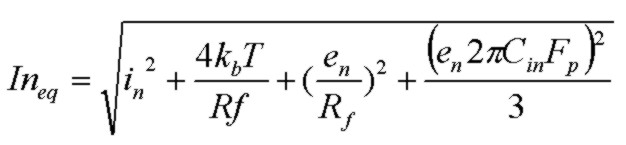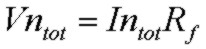(A more detailed noise calculator provides total noise (over all frequency) and cumulative noise up to any frequency.)

The last term in the In_eq expression is a result of the noise-gain zero which gains-up the op-amp noise voltage at frequencies higher than the noise-gain zero. Note that this current-density term is EXPLICITLY bandwidth dependent and can in many cases dominate the other noise current components, particularly in high-bandwidth circuits with large input capacitance and high gain (Rf). The op-amp input noise current term and the thermal noise term of Rf don't experience the same enhanced noise effect and their noise contributions start rolling off at about the pole frequency in a similar way to the transimpedance output signal voltage rolloff.
Generally speaking, to maximize transimpedance circuit sensitivity, it is desirable to use as high a gain (Rf) as possible provided there is enough signal bandwidth for the intended application and to choose an op-amp with very low input voltage noise en and input capacitance to lower the gaining-up effect. For maximum sensitivity, the goal is to select an op-amp with low en, in and Cin to achieve a noise performance in the Tz circuit which is limited only by the intrinsic thermal noise of Rf, for the particular photodiode and with the required bandwidth. The diagram below which corresponds to the default parameters in the calculator above, shows how the noise-gain curve starts to increase (gaining up the op-amps voltage noise en at a rate of 20 dB/decade) above the noise-gain's zero frequency ~ 1.7 kHz and continues to increase, raising the noise, and finally levelling off at the pole frequency 106 kHz and finally rolling off as limited by the op-amp GBW limit: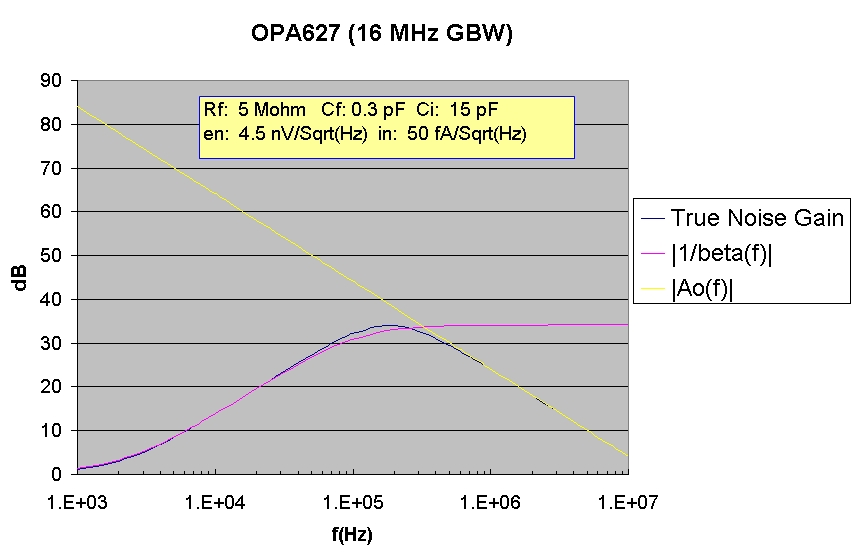For a more detailed discussion of noise considerations for transimpedance circuits, see:
The simplified noise calculation here, which assumes constant spectral densities for en and in over the bandwidth of interest, shows the size of each contributing noise current component and the total input-referred current density, total current density over the bandwidth Fp and the output total noise voltage which is In_tot*Rf. The total input-referred noise current provides a measure of the minimum detectable optical power for a photodiode receiver. A phodiode is characterized by a wavelength-dependent Responsivity (A/W) which describes the conversion efficiency of received optical power to generated photocurrent. A typical value for responsivity in the red (wavelength ~ 650 nm) part of the optical spectrum would be ~ 0.5 A/W. The optical power corresponding to the total input noise current of the transimpedance circuit is called the Noise Equivalent Power or NEP:For example, for In_tot = 27 pA, and with Responsivity = 0.5A/W, the minimum detectable optical power in this Tz circuit with the specified bandwidth would be 54 pW.

Photodiodes are typically reverse biased to reduce junction capacitance. However a biased photodiode, like any reverse biased PN junction exhibits leakage ("dark") current. This dark current introduces extra shot noise to the circuit characterized by a constant spectral density:or, using convenient units:Evaluation of this expression from the photodiode specifications will indicate if it contributes significantly to input-referred current noise. If so, it should be added to the RMS noise-current summation above. Generally smaller photodiodes will have less dark current. For example the commercial Vishay Semiconductors BPW24R Si photodiode exhibits a dark current of ~ 3nA at reverse voltages of less than 10 V. This corresponds to a shot-noise current density of ~ 31 fA/Sqrt(Hz) (or 0.03 pA/Sqrt(Hz). In the default calculator example, this is comparable to the final noise-gain term.

The photodiode dark current will also contribute to an output DC offset voltage by an amount Id*Rf or for the 5 Mohm case above and a 3nA dark current, an offset of 15 mV.

Transimpedance (Tz) Amplifier Circuit Configuration: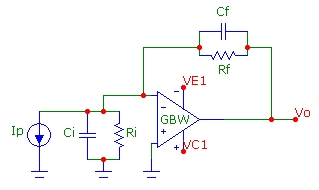Inverting (INV) Amplifier Circuit:Non-inverting (NI) Amplifier Circuit:### Generalizations:

The note above has discussed a specific feedback-network configuration. In the general case, the parallel components Rf, Cf can be replaced with an arbitrary general complex impedance Zf and the components Ri, Ci can be replaced with a general complex impedance Zi. The noise gain and feedback fraction generalize to:In this general case the same stability criterion applies regarding the intersection point fc of the noise gain and open-loop gain and the phase margin at this intersection point. Depending on the complexity of the actual network, there will be additional zeros and poles in the noise-gain function. The open-loop gain function Ao(s) of the operational amplifier will typically also have more poles at higher frequency, leading to a faster rolloff than the generic single-pole 20 dB/decade case used in the simple case above. Still, the same criterion for stability applies.